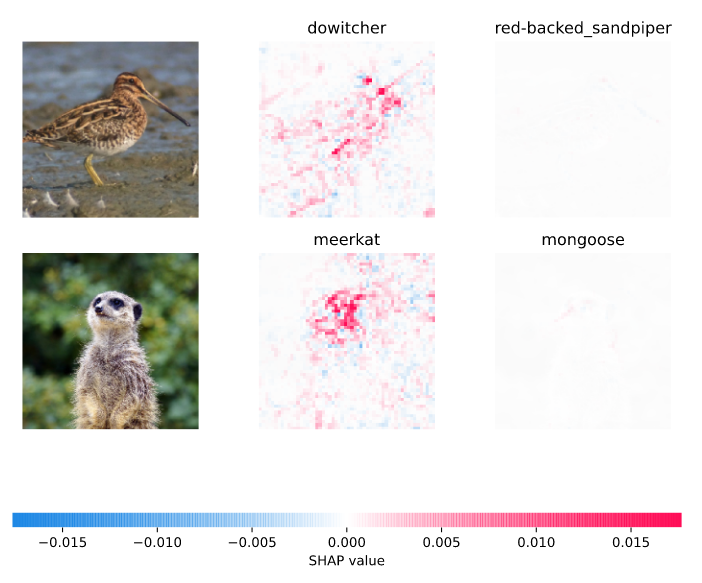# Integrate with SHAP¶

Comet integrates with SHAP.

SHAP or SHAPley Additive exPlanations is a visualization tool that can be used for making a machine learning model more explainable by visualizing its output. It can be used for explaining the prediction of any model by computing the contribution of each feature to the prediction.

## Example¶

``````# import comet_ml before shap
from comet_ml import Experiment

import shap

experiment = Experiment()

# Call any of the figure-producing functions
shap.image_plot(...)
``````

That's it for logging the figures!

To see the logged figures in the Comet UI, you can go to the Graphics tab of the Experiment View.To disable the SHAP automatic logging, set the `comet.auto_log.figures` configuration variable to `False`. For example:

``````import os
os.environ["COMET_AUTO_LOG_FIGURES"] = "0"
# SHAP auto-log of figures is now disabled

# import comet_ml before shap
from comet_ml import Experiment

import shap

experiment = Experiment()

# Call any of the figure-producing functions
shap.image_plot(...)
``````
Sep. 19, 2023